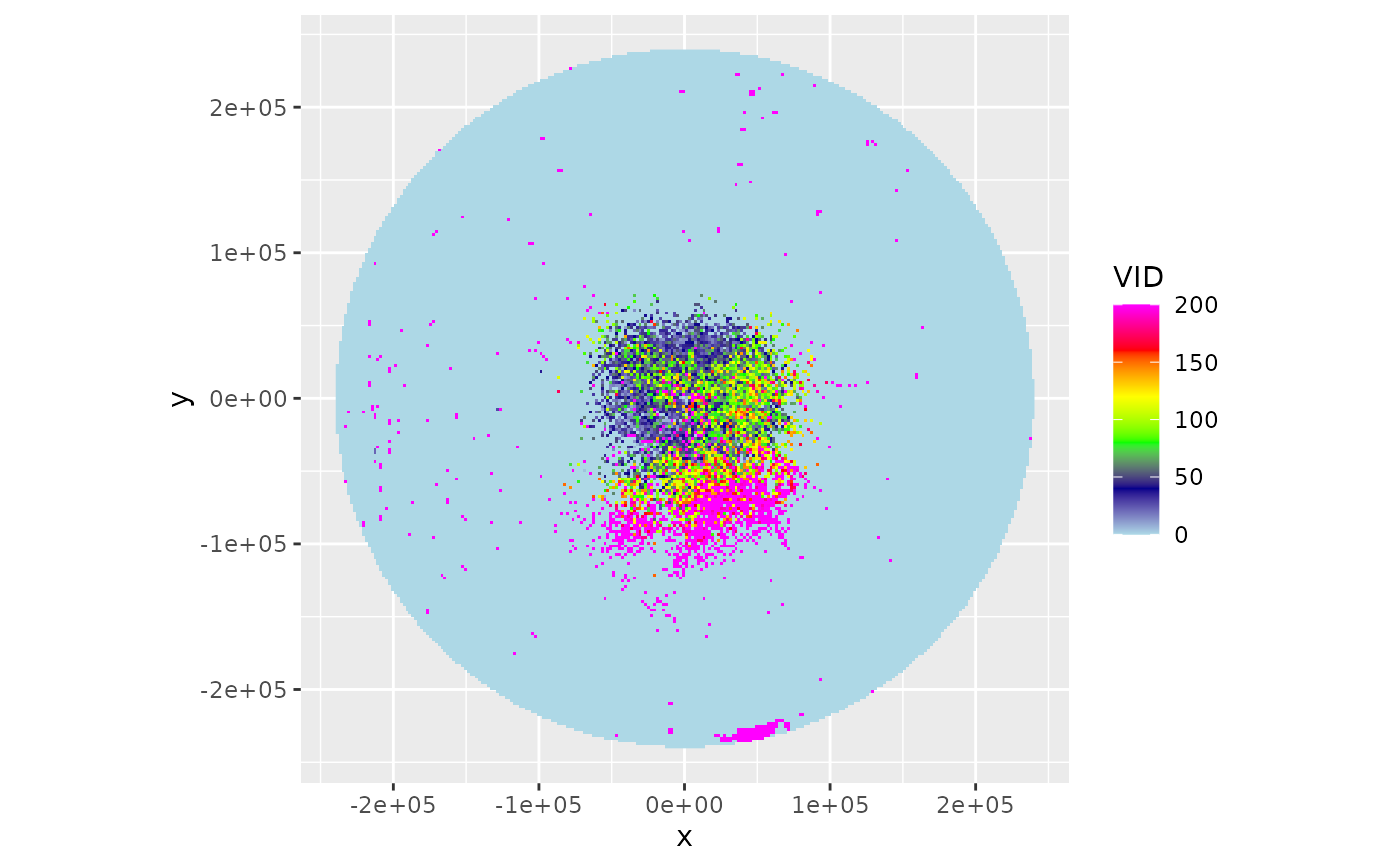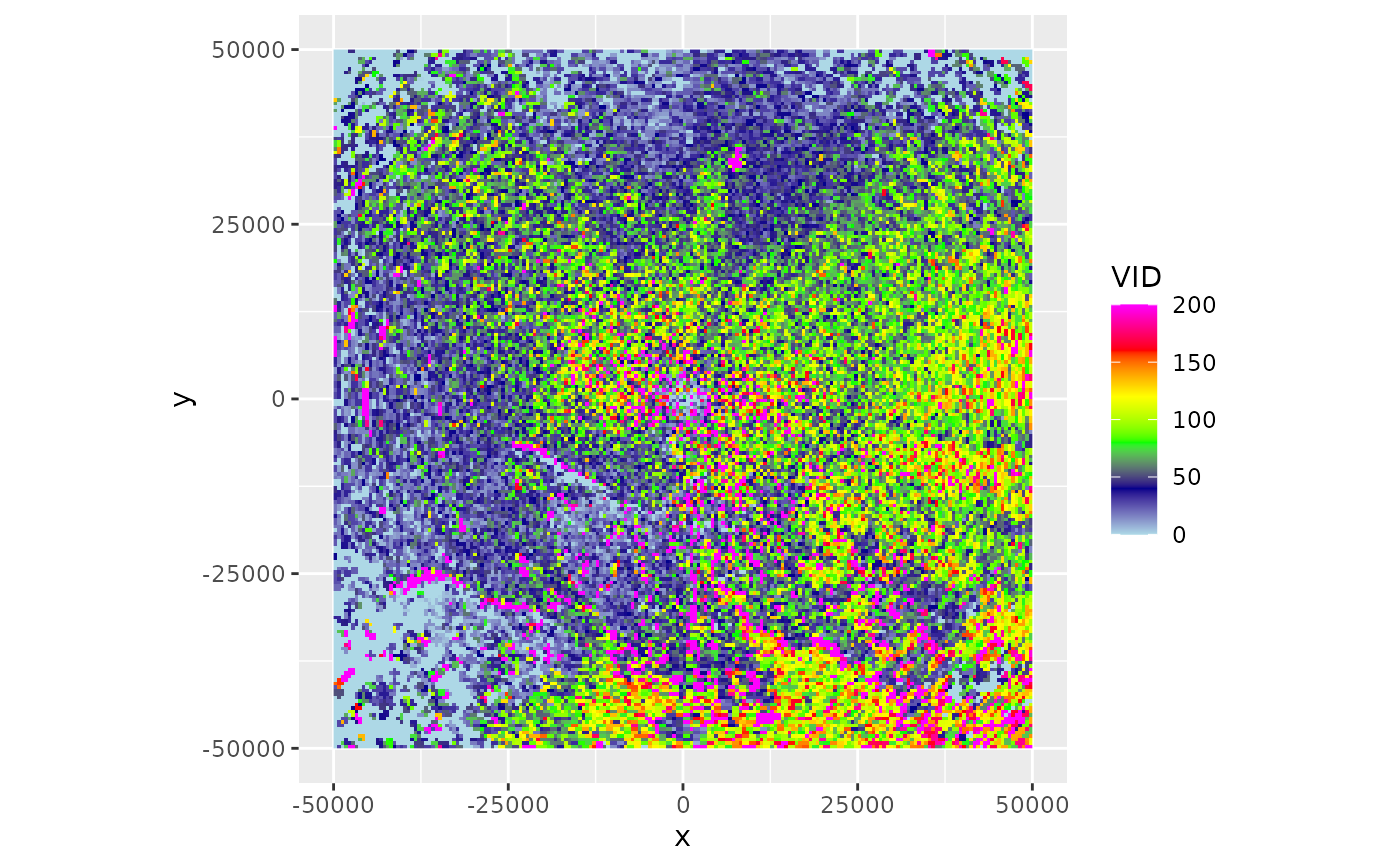This function estimates a spatial image (PPI object) of vertically integrated density (VID) based on all elevation scans of the radar, while accounting for the changing overlap between the radar beams as a function of range. The resulting PPI is a vertical integration over the layer of biological scatterers based on all available elevation scans, corrected for range effects due to partial beam overlap with the layer of biological echoes (overshooting) at larger distances from the radar. The methodology is described in detail in Kranstauber et al. (2020).

integrate_to_ppi(
pvol,
vp,
nx = 100,
ny = 100,
xlim,
ylim,
zlim = c(0, 4000),
res,
quantity = "eta",
param = "DBZH",
raster = NA,
lat,
lon,
antenna,
beam_angle = 1,
crs,
param_ppi = c("VIR", "VID", "R", "overlap", "eta_sum", "eta_sum_expected"),
k = 4/3,
re = 6378,
rp = 6357
)

## Arguments

pvol

a polar volume of class pvol

vp

a vertical profile of class vp

nx

number of raster pixels in the x (longitude) dimension

ny

number of raster pixels in the y (latitude) dimension

xlim

x (longitude) range

ylim

y (latitude) range

zlim

altitude range in meter, given as a numeric vector of length two.

res

numeric vector of length 1 or 2 to set the resolution of the raster (see res). If this argument is used, arguments nx and ny are ignored. Unit is identical to xlim and ylim.

quantity

profile quantity on which to base range corrections, 'eta' or 'dens'.

param

reflectivity factor scan parameter on which to base range corrections. Typically the same parameter from which animal densities are estimated for object vp. One of 'DBZH','DBZV','DBZ','TH','TV'.

raster

(optional) RasterLayer with a CRS. When specified this raster topology is used for the output, and nx, ny, res arguments are ignored.

lat

Geodetic latitude of the radar in degrees. If missing taken from pvol.

lon

Geodetic latitude of the radar in degrees. If missing taken from pvol.

antenna

radar antenna height. If missing taken from vp

beam_angle

numeric. Beam opening angle in degrees, typically the angle between the half-power (-3 dB) points of the main lobe

crs

character or object of class CRS. PROJ.4 type description of a Coordinate Reference System (map projection). When 'NA' (default), an azimuthal equidistant projection with origin at the radar location is used. To use a WSG84 (lat,lon) projection, use crs="+proj=longlat +datum=WGS84"

param_ppi

one or multiple of 'VIR', 'VID', 'R', 'overlap', 'eta_sum', 'eta_sum_expected'

k

Standard refraction coefficient.

re

rp

## Value

An object of class 'ppi'.

## Details

The function requires

• a polar volume, containing one or multiple scans (pvol)

• a vertical profile (of birds) calculated for that same polar volume (vp)

• a grid defined on the earth's surface, on which we will calculate the range corrected image (defined by raster, or a combination of nx,ny,res arguments).

The pixel locations on the ground are easily translated into a corresponding azimuth and range of the various scans (see function beam_range).

For each scan within the polar volume, the function calculates:

1. the vertical radiation profile for each ground surface pixel for that particular scan, using beam_profile.

2. the reflectivity expected for each ground surface pixel ($$\eta_{expected}$$), given the vertical profile (of biological scatterers) and the part of the profile radiated by the beam. This $$\eta_{expected}$$ is simply the average of (linear) eta in the profile, weighted by the vertical radiation profile.

3. the observed eta at each pixel $$\eta_{observed}$$, which is converted form DBZH using function dbz_to_eta, with DBZH the reflectivity factor measured at the pixel's distance from the radar.

For each pixel on the ground, we thus retrieve a set of $$\eta_{expected}$$ and a set of $$\eta_{observed}$$. From those we can calculate a spatial adjustment factor R as:

$$R=\sum{\eta_{observed}}/\sum{\eta_{expected}}$$, with the sum running over scans.

To arrive at the final PPI image, the function calculates

• the vertically integrated density (vid) and vertically integrated reflectivity (vir) for the profile, using the function integrate_profile.

• the spatial range-corrected PPI for VID, defined as the adjustment factor image (R), multiplied by the vid calculated for the profile

• the spatial range-corrected PPI for VIR, defined as the adjustment factor R, multiplied by the vir calculated for the profile.

If one of lat or lon is missing, the extent of the PPI is taken equal to the extent of the data in the first scan of the polar volume.

As an additional parameter, overlap between vertical profile and vertical radiation profile is calculated using beam_profile and stored as quantity overlap.

scans at 90 degree beam elevation (birdbath scans) are ignored.

## Examples

# locate example polar volume file:
pvolfile <- system.file("extdata", "volume.h5", package = "bioRad")

# load the corresponding vertical profile for this polar volume
data(example_vp)

# calculate the range-corrected ppi on a 50x50 pixel raster
my_ppi <- integrate_to_ppi(example_pvol, example_vp, nx = 50, ny = 50)
#> Warning: CRS object has comment, which is lost in output; in tests, see
#> https://cran.r-project.org/web/packages/sp/vignettes/CRS_warnings.html

# plot the vertically integrated reflectivity (VIR) using a 0-2000 cm^2/km^2 color scale:
plot(my_ppi, zlim = c(0, 2000))# \dontrun{
# calculate the range-corrected ppi on finer 2000m x 2000m pixel raster:
my_ppi <- integrate_to_ppi(example_pvol, example_vp, res = 2000)
#> Warning: CRS object has comment, which is lost in output; in tests, see
#> https://cran.r-project.org/web/packages/sp/vignettes/CRS_warnings.html

# plot the vertically integrated density (VID) using a 0-200 birds/km^2 color scale:
plot(my_ppi, param = "VID", zlim = c(0, 200))# to overlay ppi objects on a background map, first
#> Map tiles by Stamen Design, under CC BY 3.0. Data by OpenStreetMap, under ODbL.
map(my_ppi, bm)
#> Warning: CRS object has comment, which is lost in output; in tests, see
#> https://cran.r-project.org/web/packages/sp/vignettes/CRS_warnings.html# the ppi can also be projected on a user-defined raster, as follows:
# first define the raster:
template_raster <- raster::raster(raster::extent(12, 13, 56, 57), crs = sp::CRS("+proj=longlat"))

# project the ppi on the defined raster:
my_ppi <- integrate_to_ppi(example_pvol, example_vp, raster = template_raster)
#> Warning: CRS object has comment, which is lost in output; in tests, see
#> https://cran.r-project.org/web/packages/sp/vignettes/CRS_warnings.html
#> Warning: CRS object has comment, which is lost in output; in tests, see
#> https://cran.r-project.org/web/packages/sp/vignettes/CRS_warnings.html
#> Warning: CRS object has comment, which is lost in output; in tests, see
#> https://cran.r-project.org/web/packages/sp/vignettes/CRS_warnings.html
#> Warning: CRS object has comment, which is lost in output; in tests, see
#> https://cran.r-project.org/web/packages/sp/vignettes/CRS_warnings.html

# extract the raster data from the ppi object:
raster::brick(my_ppi\$data)
#> class      : RasterBrick
#> dimensions : 10, 10, 100, 6  (nrow, ncol, ncell, nlayers)
#> resolution : 0.1, 0.1  (x, y)
#> extent     : 12, 13, 56, 57  (xmin, xmax, ymin, ymax)
#> crs        : +proj=longlat +datum=WGS84 +no_defs
#> source     : memory
#> names      :          VIR,          VID,            R,      overlap,      eta_sum, eta_sum_expected
#> min values :    0.0000000,    0.0000000,    0.0000000,    0.3189387,    0.0000000,      406.9671616
#> max values : 6.052578e+04, 5.480034e+03, 6.151489e+01, 9.217694e-01, 6.468812e+04,     3.196694e+03
#>

# calculate the range-corrected ppi on an even finer 500m x 500m pixel raster,
# cropping the area up to 50000 meter from the radar.
my_ppi <- integrate_to_ppi(example_pvol, example_vp,
res = 500,
xlim = c(-50000, 50000), ylim = c(-50000, 50000)
)
#> Warning: CRS object has comment, which is lost in output; in tests, see
#> https://cran.r-project.org/web/packages/sp/vignettes/CRS_warnings.html
plot(my_ppi, param = "VID", zlim = c(0, 200))# }